## Aggregate Multivariate Distance

In reality, we have very rare of single type measurement scale. Most of cases in real measurements (especially in behavioral survey) may consist of mixed type measurement scale of nominal, ordinal, and quantitative scale. How do we handle this situation?

1. Use only normalized distance or similarity (which has value [0, 1]) for all variables.
2. Determine the weight of each feature variable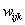(usually between 0 and 1)
3. Then, general aggregated similarity and dissimilarity index are simple weighted average of distance matrices of each features variablesandIndex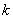represents the features variables.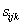and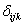are similarity and dissimilarity of between object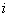and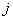for feature.

The weights are determined arbitrary, based on unit or based on the data (calibration). For example if one variable has unit ton/cubic meter and the other variable is kg/cubic meter, then weight of 1/1000 is expected to be given to give equal units. Equal weights (all= 1) for all variables may be the default weight if no other information is given.

Preferable reference for this tutorial is

Teknomo, Kardi (2019) Similarity Measurement. http:\people.revoledu.comkardi tutorialSimilarity